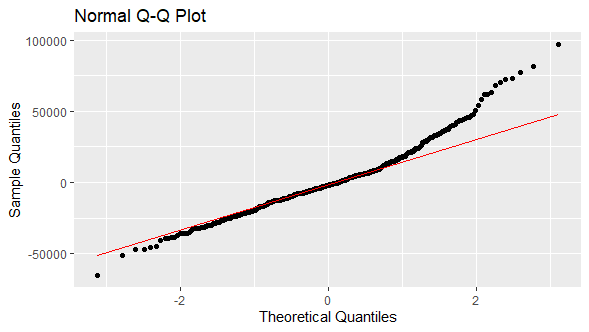# Normality in Error Term: Q-Q Plot and Jarque-Bera Test in R

Last Update: February 21, 2022

Normality in Error Term: Q-Q Plot in R can be done using `ggplot2` package `ggplot`, `stat_qq` and `stat_qq_line` functions for evaluating whether points comparing linear regression residuals sample quantiles and normal distribution theoretical quantiles are within quantiles regression line fit. Main parameters within `ggplot` function are `data` with model residuals data frame and `aes` with variables aesthetic mappings. Main parameter within `stat_qq` function is `distribution` with probability distribution quantile function to use. Main parameters within `stat_qq_line` function are `distribution` with probability distribution quantile function to use and `line.p` with percentiles vector for fitting quantiles regression line.

Normality in Error Term: Jarque-Bera Test can be done using `tseries` package `jarque.bera.test` function for evaluating whether linear regression residuals skewness and excess kurtosis are equal to zero. Main parameter within `jarque.bera.test` function is `x` with model residuals numeric vector.

As example, we can do residuals Q-Q plot and Jarque-Bera test from multiple linear regression of house price explained by its lot size and number of bedrooms using data included within `AER` package `HousePrices` object .

First, we load packages `AER` for data, `ggplot2` for Q-Q plot and `tseries` for Jarque-Bera test .

``````In :
library(AER)
library(ggplot2)
library(tseries)
``````

Second, we create `HousePrices` data object from `AER` package using `data` function and print first six rows and three columns of data using `head` function to view `data.frame` structure.

``````In :
data(HousePrices)
``````
``````Out :
price lotsize bedrooms
1 42000    5850        3
2 38500    4000        2
3 49500    3060        3
4 60500    6650        3
5 61000    6360        2
6 66000    4160        3
``````

Third, we fit multiple linear regression using `lm` function and store results within `mlr` object. Within `lm` function, parameter `formula = price ~ lotsize + bedrooms` fits model where house price is explained by its lot size and number of bedrooms.

``````In :
mlr <- lm(formula = price ~ lotsize + bedrooms, data = HousePrices)
``````

Fourth, we get residuals from `mlr` multiple linear regression object and store them within `res` object.

``````In :
res <- mlr\$residuals
``````

Fifth, we do normal Q-Q plot using `ggplot`, `stat_qq` and `stat_qq_line` functions. Within `ggplot` function, parameters `data = data.frame(res)` includes residuals as `data.frame` and `aes(sample = res)` includes residuals data variable `sample` aesthetics mapping required by `stat_qq` function. Within `stat_qq` function, parameter `distribution = qnorm` includes normal distribution quantile function. Within `stat_qq_line` function, parameters `distribution = qnorm` includes normal distribution quantile function and `line.p = c(0.25, 0.75)` includes 0.25 and 0.75 percentiles vector for fitting quantiles regression line.

``````In :
ggplot(data = data.frame(res), aes(sample = res)) +
stat_qq(distribution = qnorm) +
stat_qq_line(distribution = qnorm, line.p = c(0.25, 0.75), color = "red") +
labs(title = "Normal Q-Q Plot", x = "Theoretical Quantiles", y = "Sample Quantiles")
``````
``Out :``Figure 1. Residuals normal Q-Q plot from multiple linear regression of house price explained by its lot size and number of bedrooms.

Sixth, we do Jarque-Bera test using `jarque.bera.test` function. Within `jarque.bera.test` function, parameter `x = res` includes residuals numeric vector.

``````In :
jarque.bera.test(x = res)
``````
``````Out :
Jarque Bera Test

data:  res
X-squared = 146.85, df = 2, p-value < 2.2e-16
``````

Courses

My online courses are hosted at Teachable website.

For more details on this concept, you can view my Linear Regression in R Course.

 Data Description: Sales prices of houses sold in the city of Windsor, Canada, during July, August and September, 1987.

Original Source: Anglin, P., and Gencay, R. (1996). Semiparametric Estimation of a Hedonic Price Function. Journal of Applied Econometrics, 11, 633–648.

 AER R Package: Christian Kleiber and Achim Zeileis. (2008). Applied Econometrics with R. Springer-Verlag, New York.

ggplot2 R Package: Hadley Wickham. (2016). ggplot2: Elegant Graphics for Data Analysis. Springer-Verlag New York.

tseries R Package: Adrian Trapletti and Kurt Hornik. (2020). tseries: Time Series Analysis and Computational Finance. R package version 0.10-48.

+